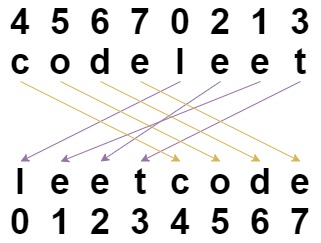# GeetCode Hub

Given a string `s` and an integer array `indices` of the same length.

The string `s` will be shuffled such that the character at the `ith` position moves to `indices[i]` in the shuffled string.

Return the shuffled string.

Example 1:```Input: s = "codeleet", `indices` = [4,5,6,7,0,2,1,3]
Output: "leetcode"
Explanation: As shown, "codeleet" becomes "leetcode" after shuffling.
```

Example 2:

```Input: s = "abc", `indices` = [0,1,2]
Output: "abc"
Explanation: After shuffling, each character remains in its position.
```

Example 3:

```Input: s = "aiohn", `indices` = [3,1,4,2,0]
Output: "nihao"
```

Example 4:

```Input: s = "aaiougrt", `indices` = [4,0,2,6,7,3,1,5]
Output: "arigatou"
```

Example 5:

```Input: s = "art", `indices` = [1,0,2]
Output: "rat"
```

Constraints:

• `s.length == indices.length == n`
• `1 <= n <= 100`
• `s` contains only lower-case English letters.
• `0 <= indices[i] < n`
• All values of `indices` are unique (i.e. `indices` is a permutation of the integers from `0` to `n - 1`).

class Solution { public String restoreString(String s, int[] indices) { } }